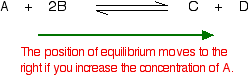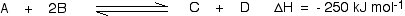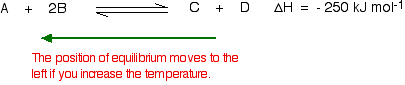# Le Chatelier's Principle Fundamentals

$$\newcommand{\vecs}{\overset { \rightharpoonup} {\mathbf{#1}} }$$ $$\newcommand{\vecd}{\overset{-\!-\!\rightharpoonup}{\vphantom{a}\smash {#1}}}$$$$\newcommand{\id}{\mathrm{id}}$$ $$\newcommand{\Span}{\mathrm{span}}$$ $$\newcommand{\kernel}{\mathrm{null}\,}$$ $$\newcommand{\range}{\mathrm{range}\,}$$ $$\newcommand{\RealPart}{\mathrm{Re}}$$ $$\newcommand{\ImaginaryPart}{\mathrm{Im}}$$ $$\newcommand{\Argument}{\mathrm{Arg}}$$ $$\newcommand{\norm}{\| #1 \|}$$ $$\newcommand{\inner}{\langle #1, #2 \rangle}$$ $$\newcommand{\Span}{\mathrm{span}}$$ $$\newcommand{\id}{\mathrm{id}}$$ $$\newcommand{\Span}{\mathrm{span}}$$ $$\newcommand{\kernel}{\mathrm{null}\,}$$ $$\newcommand{\range}{\mathrm{range}\,}$$ $$\newcommand{\RealPart}{\mathrm{Re}}$$ $$\newcommand{\ImaginaryPart}{\mathrm{Im}}$$ $$\newcommand{\Argument}{\mathrm{Arg}}$$ $$\newcommand{\norm}{\| #1 \|}$$ $$\newcommand{\inner}{\langle #1, #2 \rangle}$$ $$\newcommand{\Span}{\mathrm{span}}$$$$\newcommand{\AA}{\unicode[.8,0]{x212B}}$$

Le Chatelier's principle states that if a dynamic equilibrium is disturbed by changing the conditions, the position of equilibrium shifts to counteract the change to reestablish an equilibrium. If a chemical reaction is at equilibrium and experiences a change in pressure, temperature, or concentration of products or reactants, the equilibrium shifts in the opposite direction to offset the change. This page covers changes to the position of equilibrium due to such changes and discusses briefly why catalysts have no effect on the equilibrium position.

## Introduction

An action that changes the temperature, pressure, or concentrations of reactants in a system at equilibrium stimulates a response that partially offsets the change while a new equilibrium condition is established (2). Hence, Le Châtelier's principle states that any change to a system at equilibrium will adjust to compensate for that change. In 1884 the French chemist and engineer Henry-Louis Le Châtelier proposed one of the central concepts of chemical equilibria, which describes what happens to a system when something briefly removes it from a state of equilibrium.

It is important to understand that Le Châtelier's principle is only a useful guide to identify what happens when the conditions are changed in a reaction in dynamic equilibrium; it does not give reasons for the changes at the molecular level (e.g., timescale of change and underlying reaction mechanism).

## Concentration Changes

Le Châtelier's principle states that if the system is changed in a way that increases the concentration of one of the reacting species, it must favor the reaction in which that species is consumed. In other words, if there is an increase in products, the reaction quotient, $$Q_c$$, is increased, making it greater than the equilibrium constant, $$K_c$$. Consider an equilibrium established between four substances, $$A$$, $$B$$, $$C$$, and $$D$$:

$A + 2B \rightleftharpoons C + D$

### Increasing a concentration

What happens if conditions are altered by increasing the concentration of A?

According to Le Châtelier, the position of equilibrium will move in such a way as to counteract the change. In this case, the equilibrium position will move so that the concentration of A decreases again by reacting it with B to form more C and D. The equilibrium moves to the right (indicated by the green arrow below).In a practical sense, this is a useful way of converting the maximum possible amount of B into C and D; this is advantageous if, for example, B is a relatively expensive material whereas A is cheap and plentiful.

### Decreasing a concentration

In the opposite case in which the concentration of A is decreased, according to Le Châtelier, the position of equilibrium will move so that the concentration of A increases again. More C and D will react to replace the A that has been removed. The position of equilibrium moves to the left.This is essentially what happens if one of the products is removed as soon as it is formed. If, for example, C is removed in this way, the position of equilibrium would move to the right to replace it. If it is continually removed, the equilibrium position shifts further and further to the right, effectively creating a one-way, irreversible reaction.

## Pressure Changes

This only applies to reactions involving gases, although not necessarily all species in the reaction need to be in the gas phase. A general homogeneous gaseous reaction is given below:

$A(g) + 2B(g) \rightleftharpoons C(g) + D(g)$

### Increasing the pressure

According to Le Châtelier, if the pressure is increased, the position of equilibrium will move so that the pressure is reduced again. Pressure is caused by gas molecules hitting the sides of their container. The more molecules in the container, the higher the pressure will be. The system can reduce the pressure by reacting in such a way as to produce fewer molecules.In this case, there are three moles on the left-hand side of the equation, but only two on the right. By forming more C and D, the system causes the pressure to reduce. Increasing the pressure on a gas reaction shifts the position of equilibrium towards the side with fewer moles of gas molecules.

##### Example 1: Haber Process

$N_2 + 3H_2 \rightleftharpoons 2NH_3$

If this mixture is transferred from a 1.5 L flask to a 5 L flask, in which direction does a net change occur to return to equilibrium?

###### Solution

Because the volume is increased (and therefore the pressure reduced), the shift occurs in the direction that produces more moles of gas. To restore equilibrium the shift needs to occur to the left, in the direction of the reverse reaction.

### Decreasing the pressure

The equilibrium will move in such a way that the pressure increases again. It can do that by producing more gaseous molecules. In this case, the position of equilibrium will move towards the left-hand side of the reaction.### What happens if there are the same number of molecules on both sides of the equilibrium reaction?

In this case, increasing the pressure has no effect on the position of the equilibrium. Because there are equal numbers of molecules on both sides, the equilibrium cannot move in any way that will reduce the pressure again. Again, this is not a rigorous explanation of why the position of equilibrium moves in the ways described. A mathematical treatment of the explanation can be found on this page.

### Summary of Pressure Effects

Three ways to change the pressure of an equilibrium mixture are: 1. Add or remove a gaseous reactant or product, 2. Add an inert gas to the constant-volume reaction mixture, or 3. Change the volume of the system.

1. Adding products makes $$Q_c$$ greater than $$K_c$$. This creates a net change in the reverse direction, toward reactants. The opposite occurs when adding more reactants.
2. Adding an inert gas into a gas-phase equilibrium at constant volume does not result in a shift. This is because the addition of a non-reactive gas does not change the partial pressures of the other gases in the container. While the total pressure of the system increases, the total pressure does not have any effect on the equilibrium constant.
3. When the volume of a mixture is reduced, a net change occurs in the direction that produces fewer moles of gas. When volume is increased the change occurs in the direction that produces more moles of gas.

## Temperature Changes

To understand how temperature changes affect equilibrium conditions, the sign of the reaction enthalpy must be known. Assume that the forward reaction is exothermic (heat is evolved):In this reaction, 250 kJ is evolved (indicated by the negative sign) when 1 mole of A reacts completely with 2 moles of B. For reversible reactions, the enthalpy value is always given as if the reaction was one-way in the forward direction. The back reaction (the conversion of C and D into A and B) would be endothermic, absorbing the same amount of heat.The main effect of temperature on equilibrium is in changing the value of the equilibrium constant.

##### Temperature is Neither a Reactant nor Product

It is not uncommon that textbooks and instructors to consider heat as a independent "species" in a reaction. While this is rigorously incorrect because one cannot "add or remove heat" to a reaction as with species, it serves as a convenient mechanism to predict the shift of reactions with changing temperature. For example, if heat is a "reactant" ($$\Delta{H} > 0$$), then the reaction favors the formation of products at elevated temperature. Similarly, if heat is a "product" ($$\Delta{H} < 0$$), then the reaction favors the formation of reactants. A more accurate, and hence preferred, description is discussed below.

### Increasing the temperature

If the temperature is increased, then the position of equilibrium will move so that the temperature is reduced again. Suppose the system is in equilibrium at 300°C, and the temperature is increased 500°C. To cool down, it needs to absorb the extra heat added. In the case, the back reaction is that in which heat is absorbed. The position of equilibrium therefore moves to the left. The new equilibrium mixture contains more A and B, and less C and D.If the goal is to maximize the amounts of C and D formed, increasing the temperature on a reversible reaction in which the forward reaction is exothermic is a poor approach.

### Decreasing the temperature?

The equilibrium will move in such a way that the temperature increases again. Suppose the system is in equilibrium at 500°C and the temperature is reduced to 400°C. The reaction will tend to heat itself up again to return to the original temperature by favoring the exothermic reaction. The position of equilibrium will move to the right with more $$A$$ and $$B$$ converted into $$C$$ and $$D$$ at the lower temperature:##### Example 2

Consider the formation of water

$O_2 + 2H_2 \rightleftharpoons 2H_2O\;\;\; \Delta{H}= -125.7\, kJ$

1. What side of the reaction is favored? Because the heat is a product of the reaction, the reactants are favored.
2. Would the conversion of $$O_2$$ and $$H_2$$ to $$H_2O$$ be favored with heat as a product or as a reactant? Heat as a product would shift the reaction forward, creating $$H_2O$$. The more heat added to the reaction, the more $$H_2O$$ created

### Summary of Temperature Effects

• Increasing the temperature of a system in dynamic equilibrium favors the endothermic reaction. The system counteracts the change by absorbing the extra heat.
• Decreasing the temperature of a system in dynamic equilibrium favors the exothermic reaction. The system counteracts the change by producing more heat.

## Catalysts

Adding a catalyst makes absolutely no difference to the position of equilibrium, and Le Châtelier's principle does not apply. This is because a catalyst speeds up the forward and back reaction to the same extent and adding a catalyst does not affect the relative rates of the two reactions, it cannot affect the position of equilibrium.

However, catalysts have some application to equilibrium systems. For a dynamic equilibrium to be set up, the rates of the forward reaction and the back reaction must be equal. This does not happen instantly and for very slow reactions, it may take years! A catalyst speeds up the rate at which a reaction reaches dynamic equilibrium.

##### Example 3

You might try imagining how long it would take to establish a dynamic equilibrium if you took the visual model on the introductory page and reduced the chances of the colors changing by a factor of 1000 - from 3 in 6 to 3 in 6000 and from 1 in 6 to 1 in 6000. Starting with blue squares, by the end of the time taken for the examples on that page, you would most probably still have entirely blue squares. Eventually, though, you would end up with the same sort of patterns as before - containing 25% blue and 75% orange squares.

## Problems

1. Varying Concentration
What will happen to the equilibrium when more 2SO2 (g) is added to the following system?

$2SO_2(g) + O_2(g) \rightleftharpoons 2SO_3 (g)$

Solution:
Adding more reactants shifts the equilibrium in the direction of the products; therefore, the equilibrium shifts to the right.
Overall, the concentration of $$2SO_2$$ from initial equilibrium to final equilibrium will increase because only a portion of the added amount of $$2SO_2$$ will be consumed.
The concentration of $$O_2$$ will decrease because as the equilibrium is reestablished, $$O_2$$ is consumed with the $$2SO_2$$ to create more $$2SO_3$$. The concentration of $$2SO_3$$ will be greater because none of it is lost and more is being generated.

2. Varying Pressure
What will happen to the equilibrium when the volume of the system is decreased?

$2SO_{2(g)} + O_{2 (g)} \rightleftharpoons 2SO_{3 (g)}$

Solution:
Decreasing the volume leads to an increase in pressure which will cause the equilibrium to shift towards the side with fewer moles. In this example there are 3 moles on the reactant side and 2 moles on the product side, so the new equilibrium will shift towards the products (to the right).

3. Varying Temperature
What will happen to the equilibrium when the temperature of the system is decreased?

$N_{2(g)} + O_{2 (g)} \rightleftharpoons 2NO_{(g)} \;\;\;\; \Delta{H} = 180.5\; kJ$

Solution
Because $$\Delta{H}$$ is positive, the reaction is endothermic in the forward direction. Removing heat from the system forces the equilibrium to shift towards the exothermic reaction, so the reverse reaction will occur and more reactants will be produced.

## References

1. Pauling, L., College Chemistry, 3rd ed., Freeman, San Francisco, CA, 1964.
2. Petrucci, R., Harwood, W., Herring, F., Madura, J., General Chemistry, 9th ed., Pearson, New Jersey, 1993.
3. www.jce.divched.org/Journal/I...2N08/p1190.pdf
4. Huddle, Benjamin P. "Conceptual Questions" on LeChatelier's Principle." J. Chem. Educ.1998 75 1175.
5. Thomsen, Volker B. E. " Le Chatelier's Principle in the Sciences." J. Chem. Educ. 2000 77 173.